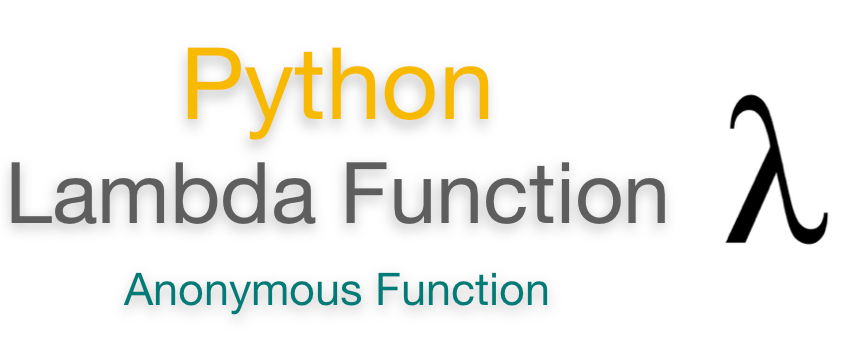top of page

# python concepts for Data Science " Lambda function "In this blog we will explain lambda function so what is the lambda function?

Python Lambda function is known as the anonymous function that is defined without a name. Python allows us to not declare the function in the standard manner by using the def keyword. Rather, the anonymous functions are declared by using the lambda keyword. However, Lambda functions can accept any number of arguments, but they can return only one value in the form of expression.

why it named lambda?

The names "lambda abstraction", "lambda function", and "lambda expression" refer to the notation of function abstraction in lambda calculus, where the usual function f(x) = M would be written (λx. M) (M is an expression that uses x). Compare to the Python syntax of lambdax:M.

The syntax of lambda function is :

`lambda arguments: expression       `

Example of lambda:

```x = lambda a:a+10
print(x)
print("sum = ",x(20))  ```
`out :`
```<function <lambda> at 0x0000019E285D16A8>
sum =  30```

Why Use Lambda Functions?

Lambda functions are used when you need a function for a short period of time. This is commonly used when you want to pass a function as an argument to higher-order functions, that is, functions that take other functions as their arguments.

The lambda function is commonly used with Python built-in functions filter() function and map() function.

* lambda with filter()

The Python built-in filter() function accepts a function and a list as an argument. It provides an effective way to filter out all elements of the sequence.

```lst = (10,22,37,41,100,123,29)
oddlist = tuple(filter(lambda x:(x%3 == 0),lst))
print(oddlist)   ```
`out:`
`(37, 41, 123, 29)`

* lambda with map()

The map() function in Python accepts a function and a list. It gives a new list which contains all modified items returned by the function for each item.

```st = (10,20,30,40,50,60)
square_list = list(map(lambda x:x**2,lst))
print(square_list)```
`out:`
`(100, 400, 900, 1600, 2500, 3600)  `

Finally, This is all about lambda function fundamental. I hope this article helped you understand the basics of the lambda function

Resources

* JavaTpoint

*W3 school